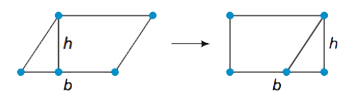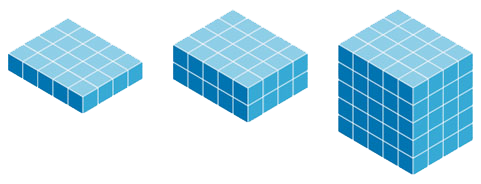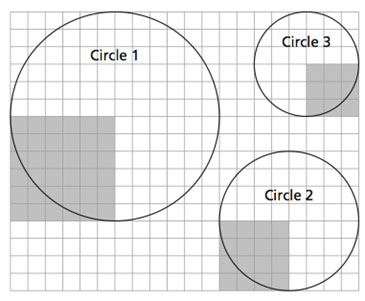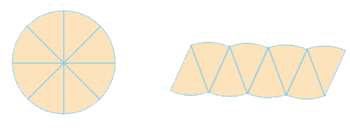Display Accessibility Tools

# Geometry and Measurement

Natural and designed objects in the world around us are outlined, supported, and decorated by an endless variety of geometric shapes. The overarching goal of the Units in the Connected Mathematics 3 (CMP3) Geometry and Measurement strand is to develop student understanding of the connections between form and function of common shapes. For example, triangles provide structural stability to bridge trusses because they are rigid figures. Hexagons are common shapes of decorative tiles, because of their many symmetries and the fact that they can be used to cover flat surfaces without overlap or gaps. Builders use 3-4-5 triangles to check perpendicularity of framing, because every such triangle must be a right triangle.

There are six Units in the Geometry and Measurement strand and connections to Geometry and Measurement topics in many Units of the other content strands. The central objectives of the strand are:

• Extend understanding and skill with geometric measurement to perimeter and area of polygons and circles and surface area and volume of right prisms, cylinders, cones, and spheres;
• Develop understanding of similarity and congruence for geometric figures, using both concepts of transformations and conditions relating side and angle measurements to establish those relationships;
• Develop proportionality connections between linear, area, and volume measurements for similar figures;
• Extend understanding of coordinate graphing to four quadrants; and
• Develop the Pythagorean Theorem for right triangles and the related distance formula for points on a coordinate grid.

The learning progressions that work toward each of those broad goals are described in the following sections.

## Extending Understanding and Skill with Geometric Measurement

Students entering the sixth grade are likely to know how to find the area of a rectangle and the volume of a rectangular prism. The sixth-grade Unit Covering and Surrounding reviews basic perimeter, area, and volume ideas, but it extends the core idea of covering (and filling) a figure with copies of a unit length (segment), area (square), or volume (cube) in several ways. First, it highlights and helps students see the difference between perimeter and area by asking “What are the dimensions of a rectangle with fixed perimeter and maximum area?” and “What are the dimensions of a rectangle with fixed area and minimum perimeter?” For example, the following visual shows two rectangles with an area of 16 square units but with different perimeters.

Area ABCD = 16 square units Area EFGH = 16 square units Perimeter ABCD = 16 units Perimeter  EFGH = 20 unitsSecond, Covering and Surrounding utilizes the principle that figures can be cut into pieces and assembled in new shapes without changing the area to develop logically the formulas for area of triangles and parallelograms. This principle is illustrated below.The area of the parallelogram on the left is the sum of two areas, a trapezoid and a triangle. The area of the rectangle on the right is also the sum of the same two areas. In both cases A = bh square units. Students are connecting a geometric idea, area, to an algebraic idea, relationships.

Finally, Covering and Surrounding lays the foundation for volume calculation in any prism by highlighting the facts that such a prism can be viewed as a stack of several layers of unit cubes and that the number of such cubes in each stack is equal to the area of the base of the prism.These same general measurement principles are applied in the Grade 7 Unit Filling and Wrapping. Filling and Wrapping extends area and volume calculation to circles, polygonal prisms, cylinders, cones, and spheres. In each case, students extend the basic ideas that apply very neatly to rectangular figures to approximation of other more complex figures. The area of a circle is approximated by covering a circle with radius squares as shown here.Area and circumference of a circle are connected by dissection. The circle below is dissected into eight sectors and then these sectors are rearranged to form an approximate parallelogram. As we dissect the circle into more and more sectors the base of the parallelogram approaches half the circumference C of the circle, and the height of the parallelogram approaches the radius r of the circle. The area of the parallelogram is approximately 0.5Cr or 0.5(2πr)r or πr2.And volume of a cylinder is calculated by applying the ‘area of base times height’ principle introduced with rectangular prisms. Surface area and volume of cylinders, cones, and spheres  is revisted in Say It With Symbols in Grade 8.

In Grade 7, Shapes and Designs reviews and extends angle measurement. Several Problems lead to fundamental results about angle measures in polygons: the derivation of the formula for exterior and interior angle measures of any polygon, and the development of the relationships among measures of angles formed by intersecting lines and parallel lines cut by transversals. Taken together, these extensions of measurement concepts and skills lay a solid foundation for development of similarity and congruence concepts in grades 7 and 8.

## Develop Understanding of Congruence and Similarity

From an informal perspective, two geometric figures are said to be congruent if they are exactly the same shape and size. Two figures are said to be similar if they are the same shape, but possibly different in size. There are at least two standard ways to make those informal understandings mathematically precise, one static and one dynamic.

The standard way of establishing congruence for figures like two triangles is to say that there is a correspondence between vertices of the figures under which corresponding pairs of sides and angles are equal in measure. The more dynamic approach to congruence is to say that there is a distance-preserving transformation (reflection, rotation, translation, or glide reflection) that maps one figure exactly on top of the other.

The static criterion for similarity says that two triangles are similar if there is a correspondence between vertices such that corresponding angles are equal in measure and the ratios of lengths for corresponding sides are equal. The more dynamic, but mathematically equivalent approach to similarity starts with the concept of dilation transformations and scale factors. For example, a dilation with scale factor 3/2 and center point P maps each point X to an image point X’, so that PX’ = 3/2PX. Then two geometric figures are similar, with scale factor k if there is some composite of dilation and rigid motion transformations that maps one figure exactly on top of the other.

From both the static and dynamic perspectives one wants to eventually arrive at answers to the question, “What minimal information about the sides and angles of two figures (especially triangles) will guarantee that they are congruent or similar?” For congruence of triangles, there are several familiar criteria—Side-Angle-Side, Side-Side-Side, and Angle-Side-Angle being most common. For similarity of triangles there are comparable results. For example, two triangles are similar if the ratios of lengths of corresponding sides are the same or the ratios of two pairs of sides are equal and the included angles are equal in measure. For similarity, there is one more criterion that highlights the significance of angle measurement: two triangles are similar if there is a correspondence between vertices so that corresponding angles are equal in measure. In fact, this criterion can be established with congruence of only two pairs of corresponding angles (because the constant angle sum of 180° in every triangle then forces the third pair of angles to be congruent).

The development of congruence and similarity in CMP3 emphasizes the more dynamic approach through transformations and it begins with the conceptually richer concept of similarity. The Grade 7 Unit Stretching and Shrinking engages students in a variety of hands-on dilation activities that stretch and shrink the size of simple figures (and connect with enlargement and reduction functions of copiers). This experience shows how size, but not shape, of dilated figures changes and how angle measures are preserved by similarity transformations.

The story of congruence and similarity, begun somewhat informally and visually in Shapes and Designs and Stretching and Shrinking, is completed in the Grade 8 unit Butterflies, Pinwheels, and Wallpaper in which the dynamic (including transformations) and static approaches come together and are applied to a variety of standard problems.

## Develop Proportionality Connections of Similar Figures

In Stretching and Shrinking, students are asked to compare ratios of side lengths in similar figures to give a visual foundation for proportional reasoning. Then comparison of perimeters and areas of similar figures introduces the fundamentally important, “How are scale factors of dilation related to changes in perimeter and area of figures?” The general principle that linear dimensions are changed by the dilation scale factor k and areas are changed by k2 is revisited and extended in subsequent measurement tasks. In Filling and Wrapping in Grade 7, students discover that if a solid figure is dilated by scale factor k, the volume is changed by factor k3.

## Extend Understanding of Coordinate Methods and the Pythagorean Theorem

The pervasive use of computer tools for graphic tasks as diverse as architectural drawing, robotic manufacturing, and movie production has made coordinate methods in geometry fundamental skills for many workers today. This trend is reflected in the CCSSM objectives for middle grades mathematics, and in the CMP3 geometry Units that meet those expectations.

The first Unit of Grade 6, Prime Time, asks students to plot factor pairs to find the visual pattern in those numbers. A related task in Covering and Surrounding asks students to display the patterns of lengths and widths that give constant area but different perimeter and constant perimeter but different area. These connections to algebraic relationships of variables are explored again in Variables and Patterns, which begins the focus on expressions and functions in the Algebra and Functions strand. Variables and Patterns and Accentuate the Negative in early Grade 7 extend graphing to all four quadrants.

Students are able to find distances on coordinate grids using informal methods in Covering and Surrounding and formal methods in Looking for Pythagoras. The latter Unit develops the Pythagorean Theorem and the standard distance formula. In addition, in Looking for Pythagoras, students develop the equation of a circle, a geometry idea expressed algebraically. Coordinates and the distance formula come together again in Butterflies, Pinwheels and Wallpaper, to develop coordinate rules for congruence and similarity transformations.

## Connections

In the spirit of Connected Mathematics, those geometric ideas and methods are applied and enhanced by work on Problems in many other Units. The most prominent and powerful connection of Geometry and Measurement to other strands of the CMP3 curriculum is the interplay of rational number and proportionality concepts in the Number and Operations strand with similarity. However, since the CMP3 approach to algebra emphasizes functional relationships between quantitative variables, the geometric methods of coordinate graphing are also prominent in every algebra Unit. That visualization of relationships between variables is also central to our development of the mathematical modeling theme in data analysis, and area models provide strong support for work with fractions, decimals, and probability. Lastly, concepts of geometry and measurement throughout CMP3 provide invaluable visual resources for problem solving and abstract reasoning about all facets of the mathematics.